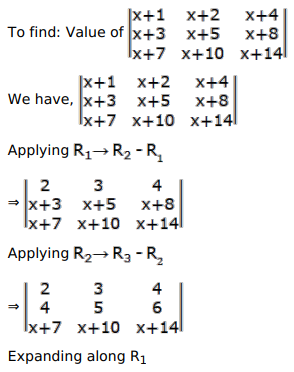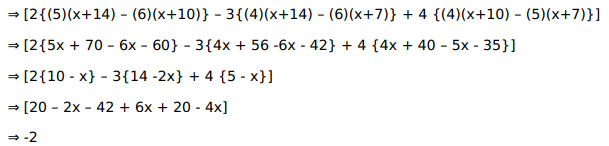# Mark the tick against the correct answer in the following:Question:

Mark the tick against the correct answer in the following:

$\left|\begin{array}{ccc}x+1 & x+2 & x+4 \\ x+3 & x+5 & x+8 \\ x+7 & x+10 & x+14\end{array}\right|=?$

A. $-2$

B. 2

C. $x^{2}-2$

D. $x^{2}+2$

Solution: